Home > INT3 > Chapter 7 > Lesson 7.1.2 > Problem7-26

7-26.
1. How do ratios of logs in different bases compare? Homework Help ✎

1. What is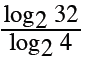?

2. What is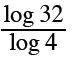?

3. What do you notice about your answers for parts (a) and (b)? Use the change of base formula to explain your results.

4. Change log2(7) into a logarithmic expression using a base of 5.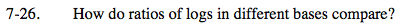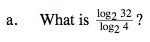Use your calculator to evaluate this expression.

$\frac{5}{2} \text{ or }\ 2.5$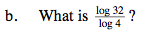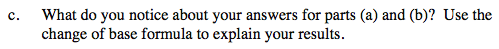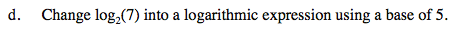Start with the definition of the logarithm.
log27 = x
2x = 7

Take the log base 5 of both sides.
log5(2x) = log57

Use the Power Property of Logarithms.
x(log52) = log57

$\log_{2}7 =\frac{\log_{5}7}{\log_{5}2}$

Notice that this answer shows a very quick way to change bases.